Courses

# Mechanism - MCQ Test 1

## 20 Questions MCQ Test RRB JE for Mechanical Engineering | Mechanism - MCQ Test 1

Description
This mock test of Mechanism - MCQ Test 1 for Mechanical Engineering helps you for every Mechanical Engineering entrance exam. This contains 20 Multiple Choice Questions for Mechanical Engineering Mechanism - MCQ Test 1 (mcq) to study with solutions a complete question bank. The solved questions answers in this Mechanism - MCQ Test 1 quiz give you a good mix of easy questions and tough questions. Mechanical Engineering students definitely take this Mechanism - MCQ Test 1 exercise for a better result in the exam. You can find other Mechanism - MCQ Test 1 extra questions, long questions & short questions for Mechanical Engineering on EduRev as well by searching above.
QUESTION: 1

Solution:
QUESTION: 2

Solution:
QUESTION: 3

### The minimum number of links in a single degree-of-freedom planar mechanism with both higher and lower kinematic pairs is

Solution:

Cam and follower has 3 links where 2 are lower and 1 higher pair, it has DOF of 1.

QUESTION: 4

A planar mechanism has 8 links and 10 rotary joints. The number of degrees of freedom of the mechanism, using Grubler's criterion, is

Solution:

Whatever may be the number of links and joints Grubler's criterion applies to mechanism with only single degree freedom. Subject to the condition 3l-2j-4=0 and it satisfy this condition.
Degree of freedom is given by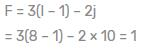QUESTION: 5

The number degrees of freedom of a planar linkage with 8 links and 9 simple revolute joints is

Solution: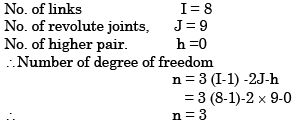QUESTION: 6

Match the approaches given below to perform stated kinematics/dynamics analysis of machine.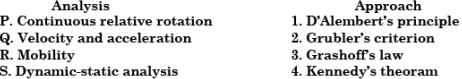Solution:

1. D` Alembert‘s principal → Dynamic-static analysis
2. Grubler’s criterion → Mobility (for plane mechanism)
3. Grashoff’s law → Continuous relative rotation
4. Kennedy’s theorem → Velocity and acceleratio

QUESTION: 7

When a cylinder is located in a Vee-block, the number of degrees of freedom which are arrested is

Solution: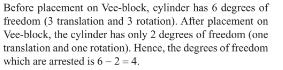QUESTION: 8

Match the following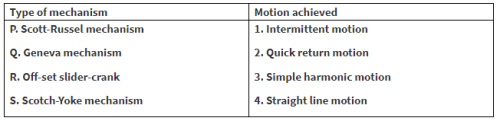Solution:
QUESTION: 9

Which of the following statements is incorrect

Solution:

It should be lesser or equal, i.e. S + L ≤ P + Q (where S and L are length of shortest and longest link, respectively).

QUESTION: 10

The mechanism used in a shaping machine is

Solution:

Quick return mechanism.

QUESTION: 11

The number of inversions for a slider crank mechanism is

Solution:

No. of links of a slider crank mechanism = 4 So there are four inversion of slider crank mechanism.

QUESTION: 12

A simple quick return mechanism is shown in the figure. The forward to return ratio of the quick return mechanism is 2: 1. If the radius of the crank O1P is 125 mm, then the distance 'd' (in mm) between the crank centre to lever pivot centre point should be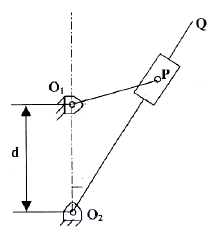Solution: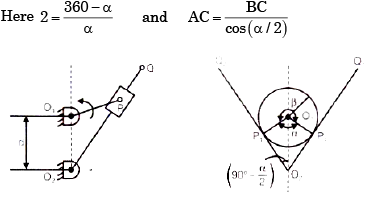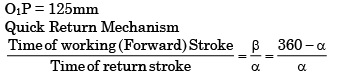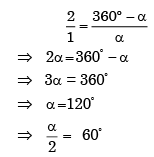The extreme position of the crank (O1P) are shown in figure.
From right triangle O2O1P1, we find that sin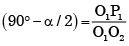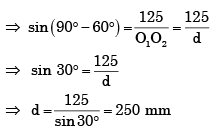QUESTION: 13

In a four-bar linkage, S denotes the shortest link length, L is the longest link length, P and Q are the lengths of other two links. At least one of the three moving links will rotate by 360° if

Solution:

According to Grashoff’s Law for a four bar mechanism, the sum of shortest and longest link lengths should not be greater than the sum of the remaining two link length.

i.e. S + L ≤ P + Q

QUESTION: 14

The lengths of the links of a 4-bar linkage with revolute pairs only are p, q, r, and s Given that p < q < r < s. Which of these links should be the fixed one, for obtaining a “double crank” mechanism?

Solution:

For Double crank mechanism Shortest link is fixed.Here shortest link is ‘P’.

QUESTION: 15

Match the following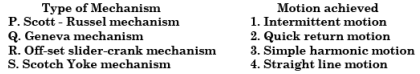Solution:
QUESTION: 16

The input link O2P of a four bar linkage is rotated at 2 rad/s in counter clockwise direction as shown below. The angular velocity of the coupler PQ in rad/s, at an instant when ∠O4O2 P = 180°, is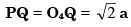and    O2P = O2O4 = a.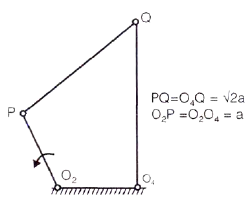Solution: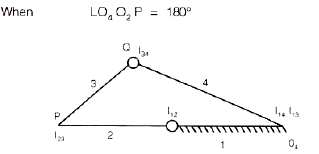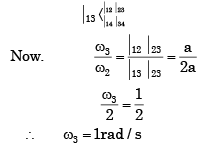QUESTION: 17

Beam engine mechanism is an example of

Solution:

Crank and lever mechanism: This is the first inversion of four bar chain. It is used to convert rotary motion of input link into oscillatory motion of output link. Beam engine mechanism is an example of this inversion and it consists of four links. The crank rotates about a fixed centre and the lever oscillates at another fixed centre.

QUESTION: 18

Two wires AO and BO support a vertical load W at O as shown in the figure below. The wires are of equal length and equal cross sectional area. The tension in each wire is equal to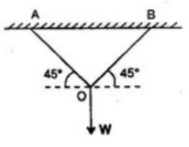Solution: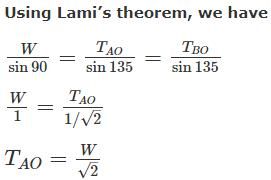QUESTION: 19

For the planar mechanism shown in figure select the most appropriate choice for the motion of link 2 when link 4 is moved upwards.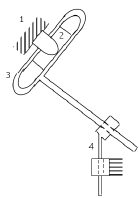Solution:

When link 4 is moved upwards, it will push link 3 upwards but since link 3&4 are joined by a revolute joint, the link will have to rotate in anticlockwise direction to move upwards. Since link 2&3 are joined, link 2 will show the same motion as link 3. So link will rotate in counter clockwise direction

QUESTION: 20

Instantaneous center of a body rolling with sliding on a stationary curved surface lies

Solution: